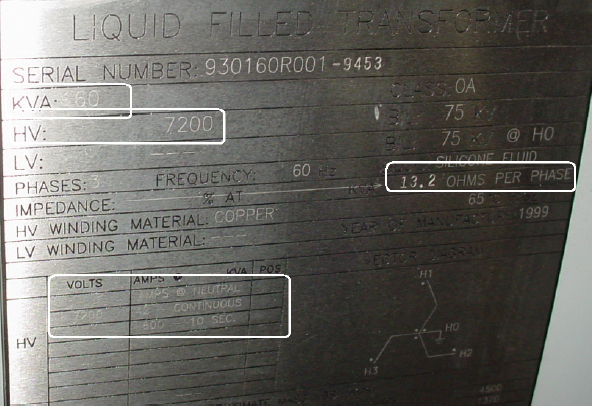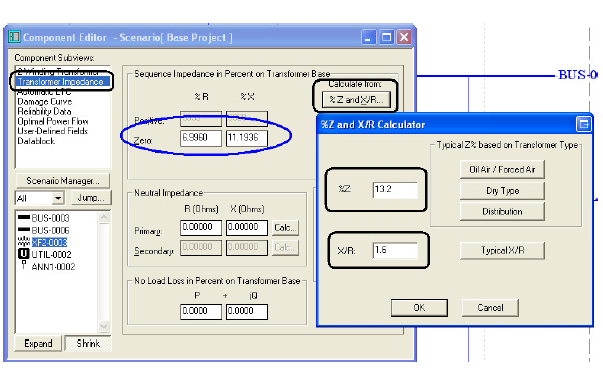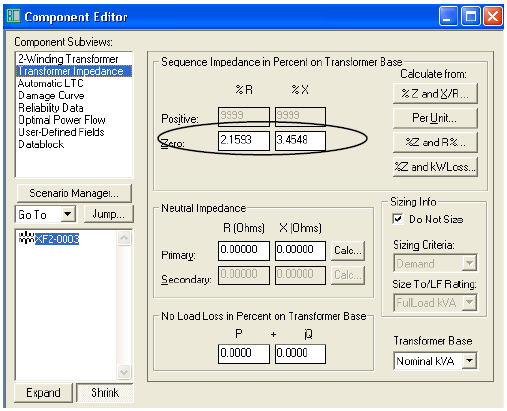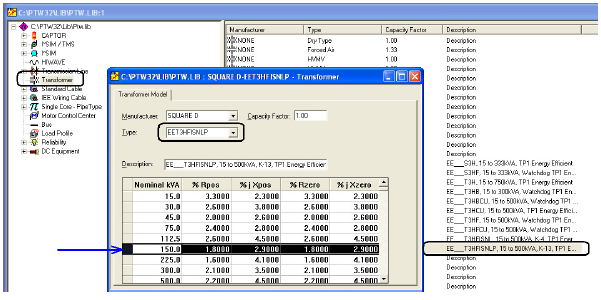Using the Nameplate data on a Zig-Zag transformer to enter the necessary input data into the Component Editor

The following picture is the nameplate of a Grounding Zig-Zag transformer:The information that we will use:

KVA rating: 160

Voltage: 7200

Ohms/phase: 13.2

Continuous Neutral amps: 42

The transformer's X/R ratio is the other piece of information that's not provided on the nameplate. This value will need to be assumed if it cannot be provided from the manufacturer.

Place a 2-winding transformer on the one-line drawing and double-click on it to bring up the Component Editor. Enter the nominal kva value (160), the connection configuration (primary is wye-grounded and the secondary is delta), and enter the primary and secondary voltages (7200 for both). If the voltages were defined for the line and load side, then those voltage will show (7200) as grayed out. Once these values are entered the full load amps value will be displayed (12.8). The Component Editor should now appear as shown:Two adjustments need to be made. Set the transformer type to Zig-Zag and adjust the Full Load KVA value. The nameplate shows the continuous neutral amps are 42. Divide this value by 3, which equals 14. This is the ampacity rating of the transformer based on its Full Load KVA rating. If this value is set at 180KVA then the ampacity of the transformer will be 14.4 amps. (See screen shot below)The information needed in the Transformer Impedance Subview, requires several steps and calculations. The first set of calculations is to solve for Ro and Xo. We will use some of the tools and calculators to accomplish this. For the first step, we use, from the nameplate, the 13.2 ohms per phase as the "raw" impedance and the transformer's X/R ratio. If we do not have this information, we will assume a ratio from the library. Look to the bottom of the FAQ for using library information to calculate a X/R ratio. In this example, we will use an X/R of 1.6. Go to the Transformer Impedance Subview and click on the %Z and X/R calculator button (see below)

Enter 13.2 (ohms) in the %Z field and 1.6 in the X/R field and click OK.

The Ro is 6.996 and the Xo is 11.1936, which is expressed in ohms.The next step is to calculate an Impedance Base (Zb). The formula is:
Zb=V*V/KVA/1000 or 7200 * 7200 / 160 /1000 = 324

Now we can express Ro and Xo in terms of per unit and in percentage. The formulas are:
Ro(pu)=Ro/Zb or 6.9960 / 324 = 0.021592593 (pu)
Xo(pu)=Xo/Zb or 11.1936 / 324 = 0.034548148 (pu)
Ro% = Ro(pu)*100 or 0.021592593 * 100 = 2.159259259 (%)
Xo% = Xo(pu)*100 or 0.034548148 * 100 = 3.454814815 (%)

The Ro% and the Xo% is the information used to enter in the Transformer Impedance Subview. In the case, they are 2.1593 and 3.4548 (% rounded).This completes the data entry of the Grounding Zig-Zag Transformer.

Using library information to calculate a "SWAG" X/R ratio

Open the PTW library and go to the Transformer section. Choose a two-winding transformer that has characteristics close to the unit that is being defined. In the Example above, a Square D unit was chosen from the Library.Since there is no 160 kVA rating, use the closest one of 150. The X/R ratio is % j Xzero dived by % Rzero (2.9 / 1.8). This results in a value of 1.6, which was used in the example above.